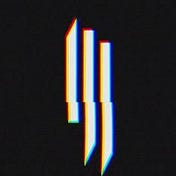# Encoded String(DECODEIT)Solution — Codechef JanuaryLong Challenge

Problem Statement

An encoder encodes the first 16 lowercase English letters using 4 bits each. The first bit (from the left) of the code is 0 if the letter lies among the first 8 letters, else it is 1, signifying that it lies among the last 8 letters. The second bit of the code is 0 if the letter lies among the first 4 letters of those 8 letters found in the previous step, else it’s 1, signifying that it lies among the last 4 letters of those 8 letters. Similarly, the third and the fourth bit each signify the half in which the letter lies.

For example, the letter j would be encoded as :

• Among (a,b,c,d,e,f,g,h || i,j,k,l,m,n,o,p), j appears in the second half. So the first bit of its encoding is 1.
• Now, among (i,j,k,l || m,n,o,p), j appears in the first half. So the second bit of its encoding is 0.
• Now, among (i,j || k,l), j appears in the first half. So the third bit of its encoding is 0.
• Now, among (i || j), j appears in the second half. So the fourth and last bit of its encoding is 1.

So j’s encoding is 1001,

Given a binary encoded string S, of length at most 105, decode the string. That is, the first 4 bits are the encoding of the first letter of the secret message, the next 4 bits encode the second letter, and so on. It is guaranteed that the string’s length is a multiple of 4.

# Input:

• The first line of the input contains an integer T, denoting the number of test cases.
• The first line of each test case contains an integer N, the length of the encoded string.
• The second line of each test case contains the encoded string S.

# Output:

For each test case, print the decoded string, in a separate line.

# Constraints

• 1≤T≤10
• 4≤N≤105
• The length of the encoded string is a multiple of 4.
• 0≤Si≤1

• 100 points : Original constraints.

# Sample Input:

`34000080000111141001`

`aapj`

# Explanation:

• Sample Case 1 :

The first bit is 0, so the letter lies among the first 8 letters, i.e., among a,b,c,d,e,f,g,h. The second bit is 0, so it lies among the first four of these, i.e., among a,b,c,d.

The third bit is 0, so it again lies in the first half, i.e., it’s either a or b. Finally, the fourth bit is also 0, so we know that the letter is a.

• Sample Case 2 :

Each four bits correspond to a character. Just like in sample case 1, 0000 is equivalent to aa. Similarly, 1111 is equivalent to p. So, the decoded string is ap.

• Sample Case 3 :

The first bit is 1, so the letter lies among the last 8 letters, i.e., among i,j,k,l,m,n,o,p. The second bit is 0, so it lies among the first four of these, i.e., among i,j,k,l.

The third bit is 0, so it again lies in the first half, i.e., it’s either i or j. Finally, the fourth bit is 1, so we know that the letter is j.

Code (Solution)

The code has been implemented in Java.

`import java.util.*;import java.lang.*;import java.io.*;class Codechef{ public static void main (String[] args) throws Exception {  BufferedReader br=new BufferedReader(new InputStreamReader(System.in));  int n=Integer.parseInt(br.readLine());  while(n--!=0){      int size=Integer.parseInt(br.readLine());      String str=br.readLine();            String s="";      String ans="";      for(int i=0;i<size;i=i+4){          if((i+4)==size)              s=str.substring(i);          else              s=str.substring(i,i+4);                       int start=0;         int mid=0;                 String arr="abcdefghijklmnop";        int end=arr.length();        String a="";        for(int j=0;j<s.length();j++){                        if(s.charAt(j)=='0'){                mid=(start+end)/2;                a=arr.substring(start,mid);                end=mid;            }            else{                mid=(start+end)/2;                if(end==16)                    a=arr.substring(mid);                else                    a=arr.substring(mid,end);                start=mid;            }        }        ans=ans+a;      }      System.out.println(ans);  } }}`

Hope you would have liked the article. Please give 50 clap to this article and follow me for more future programming related blogs.

References

https://www.codechef.com/JAN21C/problems/DECODEIT

--

--Software Engineer , Having my degree B.Tech in Information Technology from BVCOE, New Delhi. Love new Technologies. Electronic Dance Music is love.